Courses

# Test: Kinematics of Flow & Ideal Flow - 2

## 10 Questions MCQ Test Topicwise Question Bank for Mechanical Engineering | Test: Kinematics of Flow & Ideal Flow - 2

Description
This mock test of Test: Kinematics of Flow & Ideal Flow - 2 for Mechanical Engineering helps you for every Mechanical Engineering entrance exam. This contains 10 Multiple Choice Questions for Mechanical Engineering Test: Kinematics of Flow & Ideal Flow - 2 (mcq) to study with solutions a complete question bank. The solved questions answers in this Test: Kinematics of Flow & Ideal Flow - 2 quiz give you a good mix of easy questions and tough questions. Mechanical Engineering students definitely take this Test: Kinematics of Flow & Ideal Flow - 2 exercise for a better result in the exam. You can find other Test: Kinematics of Flow & Ideal Flow - 2 extra questions, long questions & short questions for Mechanical Engineering on EduRev as well by searching above.
QUESTION: 1

Solution:
QUESTION: 2

### Which of the following statement is not correct pertaining to source?

Solution: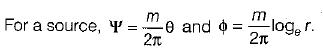Thus, the streamlines are radial lines from the source and the equipotential lines are the concentric circles with radius r = e2πφ/m.

QUESTION: 3

### An incompressible fluid flows radially outward from a line source in a steady manner. How does the velocity in any radial direction?

Solution: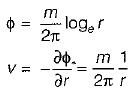∴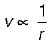QUESTION: 4

Tho doublet is a special case of source and sink pair when:

Solution:
QUESTION: 5

The potential lines in case of a doublet are

Solution:

Streamlines of a doublet are a family of circles tangent to the x-axis and with their centers on the y-axis. The equipotential lines are a family of circles tangent to the y-axis and with their centers on the x-axis.

QUESTION: 6

Consider the following statements:
1. In a source,-equipotential lines are circles.
2. Flownet is a representation of 2-D irrotational flow of incompressible fluid.
3. Boundary acts as limiting equipotential lines - in a flownet.
4. In uniform region, streamlines will be parallel and equidistant.

Which of the above are correct?

Solution:
QUESTION: 7

Match List - I (Phenomenon) with List - ll (Condition) the following: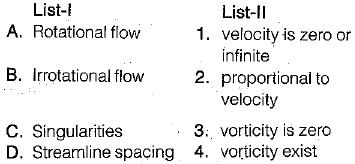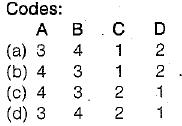Solution:
QUESTION: 8

The genera! form of expression for the continuity equationin a cartesian co-ordinate system for incompressible or compressible flow is given by

Solution:

The general form of expression for the continuity equation in a Cartesian co-ordinate system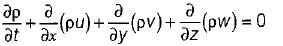QUESTION: 9

The continuity equation represents conservation of

Solution:

The continuity equation represents the conservation of mass.
Continuity equation is given by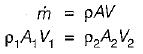QUESTION: 10

Stream line, path line and streak line are identical when the

Solution: